# Multiplication, Addition and Total Probability Rules

The additional rule determines the probability of atleast one of the events occuring.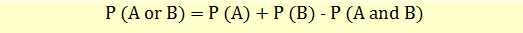If A and B are mutually exclusive, then P(A and B) = 0, so the rule can be simplified as follows: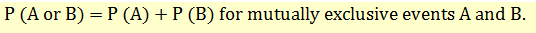### Multiplication Rule

Multiplication rule determines the joint probability of two events.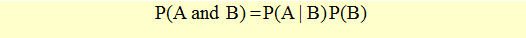Joint probability of A and B is equal to the probability of A given B multiplied by the probability of B.

If A and B are independent, then P (A/B) = P (A)and the multiplication rule simplifies to: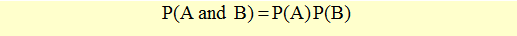### Total Probability Rule

The total probability rule determines the unconditional probability of an event in terms of probabilities conditional on scenarios.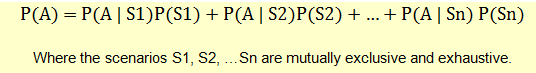Let’s take an example to understand this.

Event A: Company X’s stock price will rise.

Event B: Inflation will fall. P(B) = 0.6. Therefore, probability of inflation not falling, P(BC) = 0.4

Probability of stock price rising given a fall in inflation, P(A|B) = 0.8

Probability of stock price rising given no fall in inflation, P(A|BC) = 0.6

We can use the total probability rule to calculate the probability of a rise in stock price as follows:This is the total probability of event A occuring under all scenarios.